coding……

# Java编程拾遗『泛型——通配符&约束』

## 1. 泛型通配符

### 1.1 通配符的子类型限定

``````public <E extends T> boolean hasEqualContent(Pair<E> other) {
return new BigDecimal(getFirst().toString()).compareTo(new BigDecimal(other.getFirst().toString())) == 0
&& new BigDecimal(getSecond().toString()).compareTo(new BigDecimal(other.getSecond().toString())) == 0;
}``````

``````public boolean hasEqualContent(Pair<? extends T> other) {
return new BigDecimal(getFirst().toString()).compareTo(new BigDecimal(other.getFirst().toString())) == 0
&& new BigDecimal(getSecond().toString()).compareTo(new BigDecimal(other.getSecond().toString())) == 0;
}
``````

Pair<? extends T>表示任何泛型Pair类型，它的类型参数是传入的T的子类。比如T传入的是Number，即Pair<Number>，那么Pair<? extends T>可以是Pair<Number>、Pair<Double>，但不能是Pair<String>。通过这样的通配符类型限定，达到使用非固定通配符的效果。可以如下方式调用：

``````public class GenericTest {
public static void main(String[] args) {
Pair<Number> numberPair = new Pair<>(1.0, 2);
Pair<Integer> integerPair = new Pair<>(1, 2);
System.out.println(numberPair.hasEqualContent(integerPair));
}
}``````

• <T extends E>用于定义类型变量，它声明了一个类型变量T，可放在泛型类定义中类名后面、泛型方法返回值前面。
• <? extends E>用于实例化类型参数，它用于实例化泛型变量，?是个具体的类型，只是这个具体类型是未知的，只知道它是E或E的某个子类型。

``````public <E extends T> boolean hasEqualContent(Pair<E> other);
public boolean hasEqualContent(Pair<? extends T> other);``````

``````Pair<Integer> integerPair = new Pair<>(1, 2);
Pair<? extends Number> extendNumberPair = integerPair; //OK
extendNumberPair.setFirst(3.33); //编译报错``````

``````? extends Number getFist();
void setFirst(? extends Number);``````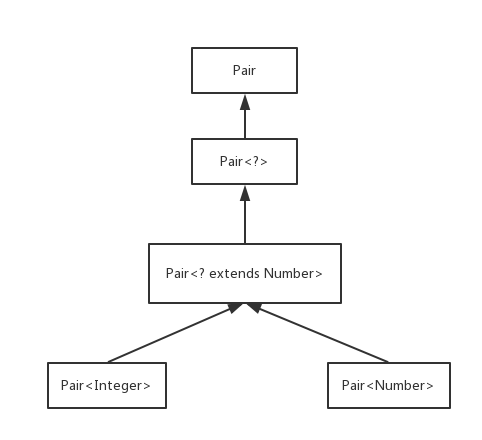### 1.2 通配符的超类型限定

``? super E``

``````public void copyTo(Pair<T> destPair) {
destPair.setFirst(this.getFirst());
destPair.setSecond(this.getSecond());
}``````

``````Pair<Integer> srcIntegerPair = new Pair<>(1, 2);
Pair<Integer> destIntegerPair = new Pair<>();
srcIntegerPair.copyTo(destIntegerPair);``````

``````Pair<Number> numberPair = new Pair<>();
Pair<Integer> integerPair = new Pair<>(1, 2);
integerPair.copyTo(numberPair); //编译报错``````

``````public void copyTo(Pair<? super T> destPair) {
destPair.setFirst(this.getFirst());
destPair.setSecond(this.getSecond());
}``````

``````Pair<Integer> integerPair = new Pair<>(1, 2);
Pair<? super Integer> superIntegerPair = integerPair; //OK
superIntegerPair.setFirst(3); //OK
Object first = superIntegerPair.getFirst(); //OK, 但是只能用Object接收
System.out.println();``````

``````public static <T extends Comparable<T>> Pair<T> minMax(T[] array) {
if (array == null || array.length == 0) {
return null;
}

T min = array;
T max = array;
for (int i = 1; i < array.length; i++) {
if (min.compareTo(array[i]) > 0) {
min = array[i];
}

if (max.compareTo(array[i]) < 0) {
max = array[i];
}
}
return new Pair<>(min, max);
}``````

``````public class Base implements Comparable<Base> {
private Integer score;

public Base(Integer score) {
this.score = score;
}

public Integer getScore() {
return score;
}

@Override
public int compareTo(Base o) {
if (this.getScore() < o.getScore()) {
return -1;
} else if (this.getScore() > o.getScore()) {
return 1;
} else {
return 0;
}
}

@Override
public String toString() {
return "Base{" +
"score=" + score +
'}';
}
}``````

``````public class Child extends Base {
public Child(int score) {
super(score);
}
}``````

``````public static void main(String[] args) {
Base base0 = new Base(3);
Base base1 = new Base(5);
Base base2 = new Base(1);
Base[] bases = new Base[]{base0, base1, base2};
Pair<Base> baseMinMaxPair = ArrayAlg.minMax(bases);
System.out.println(baseMinMaxPair);
}``````

``Pair{first=Base{score=1}, second=Base{score=5}}``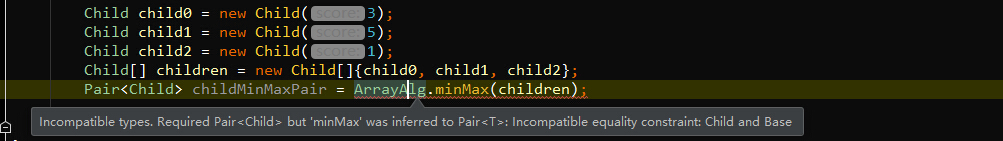``public static <T extends Comparable<? super T>> Pair<T> minMax(T[] array)``

``````public boolean hasEqualContent(Pair<? extends T> other)

public <E extends T> boolean hasEqualContent(Pair<E> other)
``````

``````//合法
public void copyTo(Pair<? super T> destPair)
//但是没有下面这种形式的限定!!!
public <E super T>void copyTo(Pair<E> destPair)
``````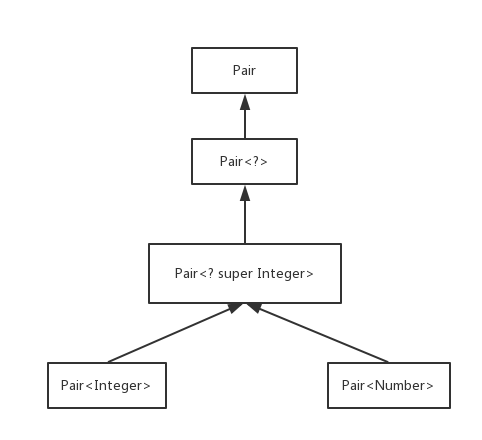### 1.3 无限定通配符

Java中还存在一种通配符类型，无限定通配符。例如，Pair<?>。看起来，这好像与原始的Pair类型一样。实际上，有很大的不同。类型Pair<?>的方法可以想像为如下形式：

``````? getFirst();
void setFirst(?);``````

getFirst的返回值只能赋给一个Object类型的引用。setFirst方法不能被调用，甚至不能用Object调用(无限定通配符是只读的)。Pair<?>和Pair的本质区别在于：可以用任意的Object对象调用原始的Pair类的set方法。

``````public static boolean hasNulls(Pair<?> p) {
return p.getFirst() == null || p.getSecond() == null;
}``````

``public static <T> boolean hasNulls(Pair<T> p);``

``````public static void swap(Pair<?> p) {
? t = p.getFirst(); // Error, 通配符?不是一个类型
p.setFirst(p.getSecond());
p.setSecond(t);
}``````

``````public static <T> void swapHelper(Pair<T> p) {
T t = p.getFirst();
p.setFirst(p.getSecond());
p.setSecond(t);
}``````

swapHelper是个泛型方法，而swap不是，它具有固定的Pair<?>类型的参数。然后，就可以swap调用swapHelper：

``````public static void swap(Pair<?> p) {
swapHelper(p);
}``````

swap可以调用swapHelper，而带类型参数的swapHelper可以写入。Java容器类中也有类似这样的用法，公共的API是通配符形式，形式更简单，但内部调用带类型参数的方法。下面总结一下通配符的使用：

• <?>和<? extends E>用于实现更为灵活的读取，它们可以用类型参数的形式替代，但通配符形式更为简洁。
• <? super E>用于实现更为灵活的写入和比较，不能被类型参数形式替代。

## 2. 泛型类的继承规则

``````Pair<Number> numberPair = new Pair<>();
Pair<Integer> integerPair = new Pair<>();

//假如下面这行是合法的
numberPair = integerPair；

/*numberPair引用地址跟IntegerPair引用地址一致

numberPair.setFirst(1.0);``````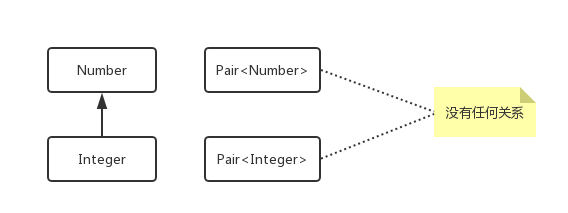``````Pair<Integer> integerPair = new Pair<>(1, 2);
Pair pair = integerPair;
pair.setFirst(1.0); //OK，编译通过
Integer first = (Integer) pair.getFirst(); //运行时异常，ClassCastException``````## 3. 泛型使用的约束

### 3.2 运行时类型检查只适用于原始类型

Java中，每个类都有一份类型信息，而每个对象也都保存着其对应类型信息的引用。这个类型信息也是一个对象，它的类型为Class，Class本身也是一个泛型类，每个类的类型对象可以通过<类名>.class的方式引用，比如String.class，Integer.class。这个类型对象也可以通过对象的getClass()方法获得，比如：

``Class<?> cls = "hello".getClass();``

``Pair<Integer>.class``

``````Pair<Integer> p1 = new Pair<Integer>(1,100);
Pair<String> p2 = new Pair<String>("hello","world");
System.out.println(Pair.class==p1.getClass());
System.out.println(Pair.class==p2.getClass());``````

instanceof是运行时判断，所以，Java中也不支持如下写法：

``if(p1 instanceof Pair<Integer>)``

``if(p1 instanceof Pair<?>)``

### 3.3 不能创建参数化类型的数组

``Pair<Integer>[] table = new Pair<Integer>[]; //Error``

``````Integer[] integerArray = new Integer;
Number[] numberArray = integerArray;
Object[] objectArray = integerArray;``````

``````Integer[] integerArray = new Integer;
Object[] objectArray = integerArray;
objectArray = "zhuoli"; //编译通过，运行时会报ArrayStoreException
``````

``````Pair<Integer>[] table = new Pair<Integer>[]; //假如可以创建，实际报错
Object[] objectArray = table;
objectArray = new Pair<String>("zhuo", "li");``````

``Pair<Integer> table = (Pair<Integer>) new Pair<?>; //编译通过``

``````List<Pair<Integer>> integerPairList = new ArrayList<>();

### 3.4 不能实例化类型变量

``````T elm = new T();
T[] arr = new T;
Class<?> clazz = T.class;``````

``````public static <T> Pair<T> makePair(Class<T> cl) {
try {
return new Pair<>(cl.newInstance(), cl.newInstance());
} catch (Exception exception) {
reutrn null;
}
}``````

``Pair<String> p = Pair.makePair(String.class);``

``````public static <T extends Comparable> T[] minMax(T[] a) {
T[] mm = new T; //Error

//……
}``````

``````public class ArrayList<E> {
private Object[] elements;

@SuppressWarnings("unchecked")
public E get(int n) {
return (E) elements[n];
}

public void set(int n, E e) {
elements[n] = e; //不需要进行转换
}
}``````

``````public static <T extends Comparable> T[] minMax(T[] a) {
Object[] mm = new Object;
//……
return (T[]) mm;
}``````

``String[] ss = minMax("Tom", "Michael", "Mary");``

``````public static <T extends Comparable> T[] minMax(T[] a) {
T[] mm = (T[]) Array.newInstance(a.getClass().getComponentType(), 2);
//……
return (T[]) mm;
}``````

``````Object[] toArray();
T[] toArray(T[] result);``````

### 3.5 泛型类类型参数不能用于静态变量和方法

``````public class Singleton<T> {

private static T instance; // Error，T为泛型类类型参数

public synchronized static T getInstance(){ //Error，T为泛型类类型参数
if(instance==null){
// 创建实例
}
return instance;
}
}    ``````

``public static <T extends Comparable<? super T>> Pair<T> minMax(T[] array);``

### 3.6 注意擦除后的冲突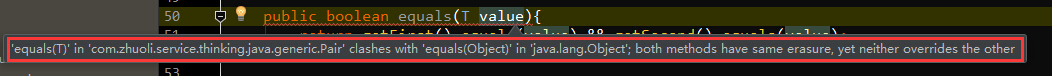``````boolean equals(String) //defined in Pair<T>
boolean equals(Object) //inherited from Object``````

``````class Base implements Comparable<Base>

class Child extends Base``````

Child没有专门实现Comparable接口，上节我们说Base类已经有了比较所需的全部信息，所以Child没有必要实现，可是如果Child希望自定义这个比较方法呢？直觉上，可以这样修改Child类：

``````class Child extends Base implements Comparable<Child>{
@Override
public int compareTo(Child o) {

}
//...
}
``````

``````class Child extends Base {
@Override
public int compareTo(Base o) {
if(!(o instanceof Child)){
throw new IllegalArgumentException();
}
Child c = (Child)o;
//...
return 0;
}
//...
}
``````

1. 《Java核心技术 卷Ⅰ》
2. 《Java编程的逻辑》
3.  java 泛型详解-绝对是对泛型方法讲解最详细的，没有之一
4. Java泛型详解

• QQ咨询
• 回顶